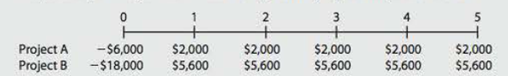Chapter 11, Problem 7PFundamentals of Financial Manageme...

14th Edition
Eugene F. Brigham + 1 other
ISBN: 9781285867977

Solutions

Chapter
SectionFundamentals of Financial Manageme...

14th Edition
Eugene F. Brigham + 1 other
ISBN: 9781285867977
Textbook Problem

CAPITAL BUDGETING CRITERIA A firm with a 14% VVACC is evaluating two projects for this year’s capital budget. After-tax cash flows, including depreciation, are as follows:a. Calculate NPV, IRR, MIRR, payback, and discounted payback for each project. b. Assuming the projects are independent, which one(s) would you recommend? c. If the projects are mutually exclusive, which would you recommend? d. Notice that the projects have the same cash flow timing pattern. Why is there a conflict between NPV and IRR?

a.

Summary Introduction

To calculate: NPV, IRR, MIRR, payback period and discounted payback period for given projects at 14% WACC.

Capital Budgeting:

It refers to the long term investment decisions that has been taken by the top management of a company and that are irreversible in nature. These decisions requires investment of large amount of cash of the company.

Net Present Value (NPV):

It is a method under capital budgeting, which includes the calculation of net present value of the project in which company is investing. The calculation is done by calculating the difference between the value of cash inflow and value of cash outflow after taking into consideration the discounted rate.

Internal Rate of Return (IRR):

It refers to the rate of return that is computed by the company to make a decision of selection of a project for investment. This rate provides the basis for selection of projects with lower cost of capital and rejection of project with higher cost of capital.

Modified Internal Rate of Return (MIRR):

It refers to the rate of return that is computed by the company to make a decision of selection and ranking of a project for investment. This is a modified version of IRR with reinvestment of cash flows at the cost of capital.

Payback Period:

It refers to the time period that is required to get an amount invested in a project with some return on it. In other words, it is the time that a project takes to repay the amount invested with some return attached to it.

Discounted Payback Period:

It refers to the time period that a project takes to repay the amount invested with some returns attached to it after considering the time value of money or discounted cash flows.

Explanation

The calculation for NPV, IRR and MIRR of project A in spreadsheet is:

Table (1)

The formula to calculate payback period is:

PaybackPeriod=(YearofLastNegativeCumulativeCashFlow)+|LastNegativeCumulativeCashflow|(PositiveCashFlowintheNextYear)

Substitute 2 for year of last negative cumulative cash flow,-$2,000 for last negative cumulative cash flow and$2,000 for positive cash flow in the next year in the above formula to calculate payback period for project A.

PaybackPeriod=(2)+|$2,000|($2,000)=2+1=3

The formula to calculate the discounted payback period is:

(DicountedPaybackPeriod)=(YearofLastNegativeCumulativePresentValue)+|LastNegativeCumulativePresentValue|(PositivePresentValueintheNextYear)

Substitute 4 for year of last negative cumulative present value,-$172.57 for last negative cumulative present value and$1,038.74 for positive present value in the next year in the above formula to calculate discounted payback period for project A:

(DicountedPaybackPeriod)=(4)+|$172.57|(1038.74)=4+0.16=4.16 The NPV is$866.16, IRR is 20%, MIRR is 17%, payback period is 3 years and discounted payback period is 4.16 years for project A.

The calculation for NPV, IRR and MIRR of project B in spreadsheet is:

b.

Summary Introduction

To explain: Whether to choose project A or project B when they are independent.

Independent Projects:

It refers to those projects, where the acceptance or rejection of one project does not have any effect on the decisions related to acceptance or rejection of any other project. In case of independent projects, two projects can be accepted simultaneously.

c.

Summary Introduction

To explain: Whether to choose project A or project B, when they are mutually exclusive.

Mutually Exclusive Projects:

It refers to the group of projects in which if one project is accepted, it will automatically implies the rejection of rest. It refers to those projects for which investment cannot be made together.

d.

Summary Introduction

To explain: The reason of conflict between the NPV and IRR.

Still sussing out bartleby?

Check out a sample textbook solution.

See a sample solution

The Solution to Your Study Problems

Bartleby provides explanations to thousands of textbook problems written by our experts, many with advanced degrees!

Get Started

Find more solutions based on key concepts